Author: Oscar Cronquist Article last updated on February 07, 2019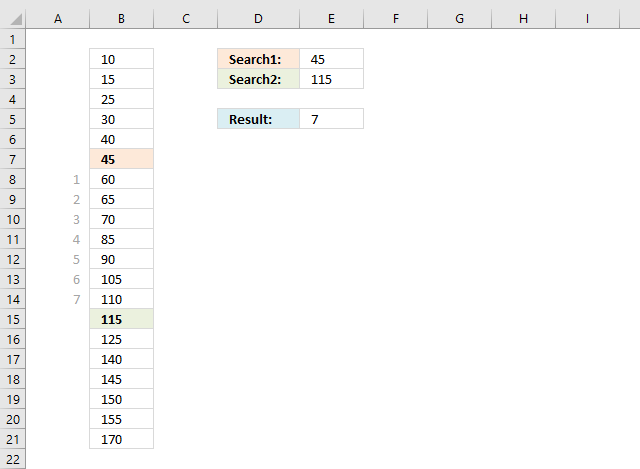This article demonstrates formulas that calculate the number of cells between two values, the first scenario involves two search values and unique numbers in column B.

If this is not what you are looking for then scroll down to see more examples.

Formula in cell E5:

=ABS(MATCH(E2,\$B\$2:\$B\$21,0)-MATCH(E3,\$B\$2:\$B\$21,0))-1

Since there is only one instance of each search value in column B you only need a small formula to calculate the number of cells between the values.

Column A shows the cell count between the two values.

### Explaining formula in cell E5

The formula finds the first value specified in cell E2 (45) in column B and returns the relative position of 45. It then continues with the second value given in cell E3.

The difference between the calculated relative positions is the number of cells between the values.

#### Step 1 - Find position of first value

The MATCH function returns the relative position of the value in cell E2 in cell range B2:B21.

MATCH(E2,\$B\$2:\$B\$21,0)

becomes

MATCH(45, {10; 15; 25; 30; 40; 45; 60; 65; 70; 85; 90; 105; 110; 115; 125; 140; 145; 150; 155; 170}, 0)

and returns 6. Value 45 is the sixth value in the array.

Note, regarding the MATCH function. Remember to use 0 (zero) as the last argument in order to perform an exact match.

#### Step 2 - Find position of second value

MATCH(E3,\$B\$2:\$B\$21,0)

becomes

MATCH(115, {10; 15; 25; 30; 40; 45; 60; 65; 70; 85; 90; 105; 110; 115; 125; 140; 145; 150; 155; 170}, 0)

and returns 14. Value 115 is the 14th value in the array.

#### Step 3 - Subtract row numbers

MATCH(E2,\$B\$2:\$B\$21,0)-MATCH(E3,\$B\$2:\$B\$21,0)

becomes

6-14

and returns -8.

#### Step 4 - Remove sign

The ABS function converts negative numbers to positive numbers.

ABS(MATCH(E2,\$B\$2:\$B\$21,0)-MATCH(E3,\$B\$2:\$B\$21,0))-1

becomes

ABS(-8)-1

becomes

8-1 and returns 7 in cell E5.

### Multiple values - two search values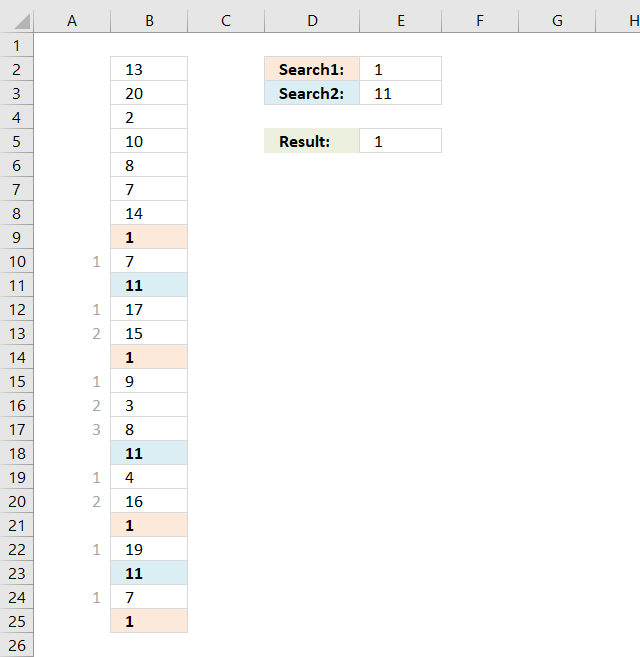The image above shows the second scenario, there are multiple instances of each search value in column B. This requires a somewhat more complicated formula to get the smallest number of cells between the two search values.

The order makes no difference, in other words, if search value 2 is found first makes no difference in this calculation.

Array formula in cell E5:

=AGGREGATE(15, 6, ABS(IF(B2:B25=E2, ROW(B2:B25), "")-TRANSPOSE(IF(B2:B25=E3, ROW(B2:B25), ""))), 1)-1

To enter an array formula, type the formula in a cell then press and hold CTRL + SHIFT simultaneously, now press Enter once. Release all keys.

The formula bar now shows the formula with a beginning and ending curly bracket telling you that you entered the formula successfully. Don't enter the curly brackets yourself.

### Explaining formula in cell E5

#### Step 1 - Calculate row numbers for first search value

The IF function has three arguments, the first one must be a logical expression. If the expression evaluates to TRUE then one thing happens (argument 2) and if FALSE another thing happens (argument 3).

IF(B2:B25=E2, ROW(B2:B25), "")

becomes

IF({13; 20; 2; 10; 8; 7; 14; 1; 7; 11; 17; 15; 1; 9; 3; 8; 11; 4; 16; 1; 19; 11; 7; 1}=1, ROW(B2:B25), "")

becomes

IF({FALSE; FALSE; FALSE; FALSE; FALSE; FALSE; FALSE; TRUE; FALSE; FALSE; FALSE; FALSE; TRUE; FALSE; FALSE; FALSE; FALSE; FALSE; FALSE; TRUE; FALSE; FALSE; FALSE; TRUE}, ROW(B2:B25), "")

becomes

IF({FALSE; FALSE; FALSE; FALSE; FALSE; FALSE; FALSE; TRUE; FALSE; FALSE; FALSE; FALSE; TRUE; FALSE; FALSE; FALSE; FALSE; FALSE; FALSE; TRUE; FALSE; FALSE; FALSE; TRUE}, {2; 3; 4; 5; 6; 7; 8; 9; 10; 11; 12; 13; 14; 15; 16; 17; 18; 19; 20; 21; 22; 23; 24; 25}, "")

and returns

{""; ""; ""; ""; ""; ""; ""; 9; ""; ""; ""; ""; 14; ""; ""; ""; ""; ""; ""; 21; ""; ""; ""; 25}.

#### Step 2 - Calculate row numbers for second search value

The TRANSPOSE function rearranges values distributed vertically to horizontally.

TRANSPOSE(IF(B2:B25=E3, ROW(B2:B25), ""))

becomes

TRANSPOSE({"";"";"";"";"";"";"";"";"";11;"";"";"";"";"";"";18;"";"";"";"";23;"";""})

and returns

{"", "", "", "", "", "", "", "", "", 11, "", "", "", "", "", "", 18, "", "", "", "", 23, "", ""}.

#### Step 3 - Subtract first array with second array

IF(B2:B25=E2, ROW(B2:B25), "")-TRANSPOSE(IF(B2:B25=E3, ROW(B2:B25), ""))

becomes

{""; ""; ""; ""; ""; ""; ""; 9; ""; ""; ""; ""; 14; ""; ""; ""; ""; ""; ""; 21; ""; ""; ""; 25}-{"", "", "", "", "", "", "", "", "", 11, "", "", "", "", "", "", 18, "", "", "", "", 23, "", ""}

and returns an array too big to show here, however, an image would work if I replace all error values with a "-".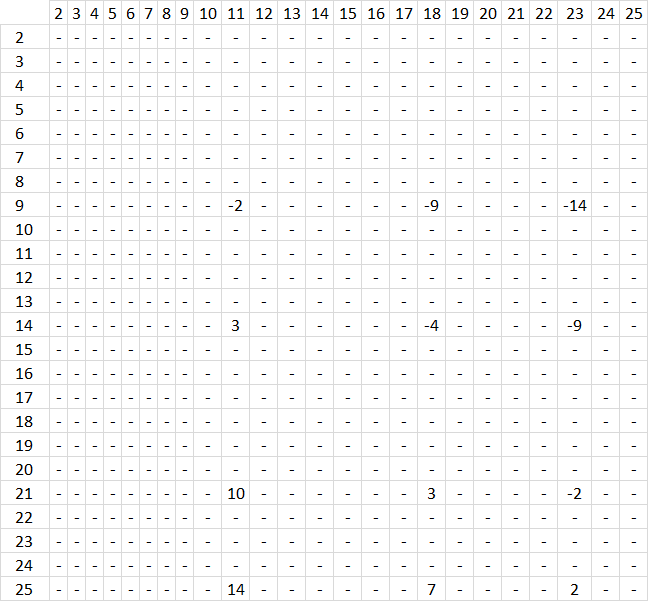What does the image above tell us? It shows row numbers horizontally and vertically. The first search value (1) has its row numbers displayed vertically, there are four instances of the search value in column B, row 9, 14, 21 and 25.

The second search value har its row numbers displayed horizontally, it is found on row 11, 18 and 23. Example, the intersection of 9 and 11 shows -2. It means that 9-11 equals -2, the whole array shows the intersection between all instances of each search value.

This allows us to extract the smallest distance between two cells, or if you like the largest distance or any in between.

#### Step 4 - Convert negative values to positive values

The ABS function removes the minus sign from negative values.

ABS(IF(B2:B25=E2, ROW(B2:B25), "")-TRANSPOSE(IF(B2:B25=E3, ROW(B2:B25), "")))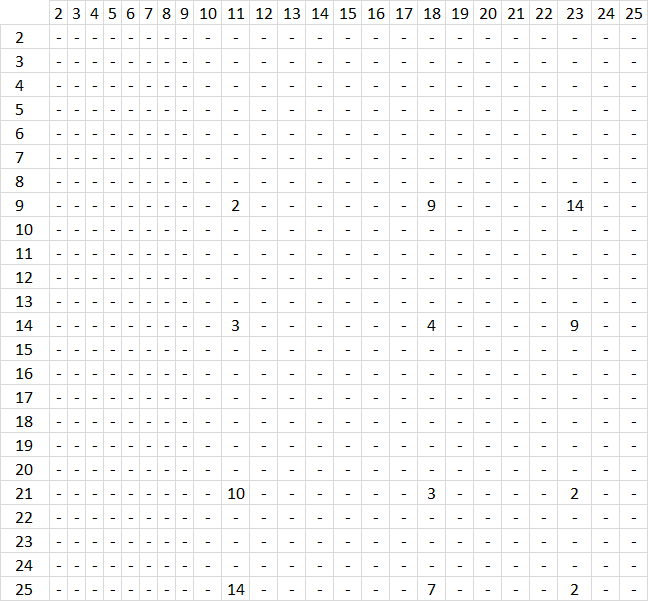#### Step 5 - Extract smallest number

The AGGREGATE function is better than the SMALL function, it also lets you ignore error values.

AGGREGATE(15, 6, ABS(IF(B2:B25=E2, ROW(B2:B25), "")-TRANSPOSE(IF(B2:B25=E3, ROW(B2:B25), ""))), 1)-1

becomes

2-1

and returns 1 in cell E5.

### Multiple values - one search value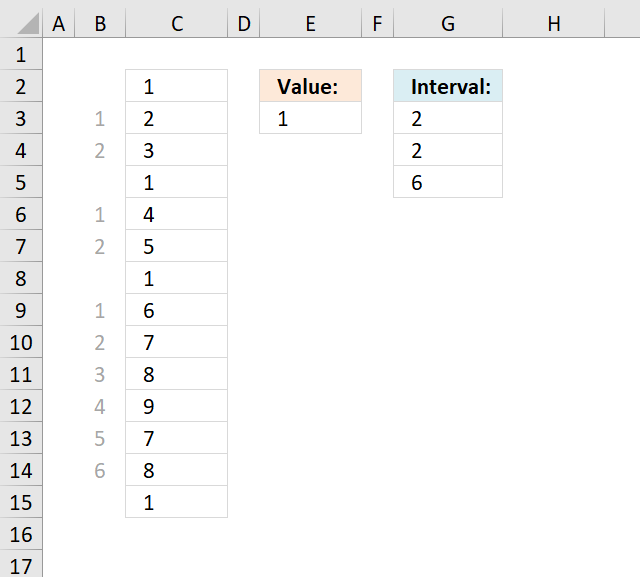I need to count in a list the interval between the same value.

Example list,

1-2-3-1-4-5-1-6-7-8-9-7-8-1

So the answer must be for the value 1 the spaces are 2,2,6.

thank you

Array Formula in cell E2:

=SMALL(IF(\$C\$2:\$C\$15=\$E\$3, MATCH(ROW(\$C\$2:\$C\$15), ROW(\$C\$2:\$C\$15)), ""), ROW(A1)+1)-SMALL(IF(\$C\$2:\$C\$15=\$E\$3, MATCH(ROW(\$C\$2:\$C\$15), ROW(\$C\$2:\$C\$15)), ""), ROW(A1))-1

How to create an array formula

1. Select cell E2
2. Type above array formula
3. Press and hold Ctrl + Shift
4. Press Enter once
5. Release all keys

How to copy array formula

1. Select cell E2
2. Copy (Ctrl + c)
3. Select cell range E3:E5
4. Paste (Ctrl + v)

### Explaining formula in cell E2

There are two parts that calculate the position of the first instance and the second instance, the formula then subtracts the positions in order to get the distance.

#### Step 1 - Get position of second instance

SMALL(IF(\$C\$2:\$C\$15=\$E\$3, MATCH(ROW(\$C\$2:\$C\$15), ROW(\$C\$2:\$C\$15)), ""), ROW(A1)+1)

becomes

SMALL(IF({TRUE; FALSE; FALSE; TRUE; FALSE; FALSE; TRUE; FALSE; FALSE; FALSE; FALSE; FALSE; FALSE; TRUE},MATCH(ROW(\$C\$2:\$C\$15),ROW(\$C\$2:\$C\$15)),""),ROW(A1)+1)

becomes

SMALL(IF({TRUE;FALSE;FALSE;TRUE;FALSE;FALSE;TRUE;FALSE;FALSE;FALSE;FALSE;FALSE;FALSE;TRUE},{1;2;3;4;5;6;7;8;9;10;11;12;13;14},""),ROW(A1)+1)

becomes

SMALL(IF({TRUE; FALSE; FALSE; TRUE; FALSE; FALSE; TRUE; FALSE; FALSE; FALSE; FALSE; FALSE; FALSE; TRUE},{1; 2; 3; 4; 5; 6; 7; 8; 9; 10; 11; 12; 13; 14},""),ROW(A1)+1)

becomes

SMALL({1;"";"";4;"";"";7;"";"";"";"";"";"";14},ROW(A1)+1)

becomes

SMALL({1;"";"";4;"";"";7;"";"";"";"";"";"";14},2)

and returns 4. The second instance of 1 is found on row 4 (C5 is on row 4 in cell range \$C\$2:\$C\$15).

#### Step 2 - Get position of first instance

SMALL(IF(\$C\$2:\$C\$15=\$E\$3, MATCH(ROW(\$C\$2:\$C\$15), ROW(\$C\$2:\$C\$15)), ""), ROW(A1))

becomes

SMALL({1;"";"";4;"";"";7;"";"";"";"";"";"";14}, ROW(A1))

becomes

SMALL({1;"";"";4;"";"";7;"";"";"";"";"";"";14}, 1)

and returns 1. The first instance of 1 is found on row 1 (C2 is on row 1 in cell range \$C\$2:\$C\$15).

#### Step 3 - Subtract positions

SMALL(IF(\$C\$2:\$C\$15=\$E\$3, MATCH(ROW(\$C\$2:\$C\$15), ROW(\$C\$2:\$C\$15)), ""), ROW(A1)+1)-SMALL(IF(\$C\$2:\$C\$15=\$E\$3, MATCH(ROW(\$C\$2:\$C\$15), ROW(\$C\$2:\$C\$15)), ""), ROW(A1))-1

becomes

4-1-1

and returns 2 in cell G3.

How to remove #num errors

=IFERROR(SMALL(IF(\$A\$1:\$A\$14=\$C\$2, MATCH(ROW(\$A\$1:\$A\$14), ROW(\$A\$1:\$A\$14)), ""), ROW(A1)+1)-SMALL(IF(\$A\$1:\$A\$14=\$C\$2, MATCH(ROW(\$A\$1:\$A\$14), ROW(\$A\$1:\$A\$14)), ""), ROW(A1))-1,"")

### Get the Excel fileList-interval-between-two-valuesv2.xlsx